Automatic Modulation Recognition in OFDM Systems using Cepstral Analysis and Support Vector Machines | OMICS International
Journal of Telecommunications System & Management
All submissions of the EM system will be redirected to Online Manuscript Submission System. Authors are requested to submit articles directly to Online Manuscript Submission System of respective journal.

# Automatic Modulation Recognition in OFDM Systems using Cepstral Analysis and Support Vector Machines

Rasha M. Al-Makhlasawy1*, Mustafa M. Abd Elnaby1, Heba A. El-Khobby1, S. El-Rabaie2 and Fathi. E. Abd. El-samie2

1Faculty of Engineering, Tanta University, Tanta, Egypt

2Faculty of Electronic Engineering, Menoufia University, Menouf, Egypt

*Corresponding Author:
Rasha M. Al-Makhlasawy
Faculty of Engineering
Tanta University, Tanta, Egypt
E-mail: [email protected]

Received date: October 30, 2012; Accepted date: November 30, 2012; Published date: December 03, 2012

Citation: Al-Makhlasawy RM, Elnaby MMA, El-Khobby HA, El-Rabaie S, El-samie FEA (2012) Automatic Modulation Recognition in OFDM Systems using Cepstral Analysis and Support Vector Machines. J Telecommun Syst Manage 1:105. doi: 10.4172/2167-0919.1000105

Copyright: © 2012 Al-Makhlasawy RM, et al. This is an open-access article distributed under the terms of the Creative Commons Attribution License, which permits unrestricted use, distribution, and reproduction in any medium, provided the original author and source are credited.

Visit for more related articles at Journal of Telecommunications System & Management

#### Abstract

This paper discusses the modulation recognition for OFDM signals in different Signal to Noise Ratio (SNR) and multipath channels. In this paper, the Mel Frequency Cepstral Coefficients (MFCCs) used for feature extraction and the Support Vector Machine (SVM) as classifier or Artificial Neural Network (ANN). Simulation results indicate that the proposed feature classifier have good performances in different SNR and multipath channels for both recognition rate and CPU time from the Artificial Neural Network (ANN), and the SVM classifier’s generalizing ability proves to be good.

#### Keywords

ADMR; MFCCs; SVM; OFDM; LMMSE; ZF

#### Introduction

Automatic modulation recognition of digital communication signals is an important signal processing problem in communications and communication related fields. It is an intermediate step between signal interception and information recovery, which automatically identifies the modulation type of the received signal for further demodulation and other tasks. One problem is how to receive the signals modulated by various modulation types optimally, which can be done by using the automatic modulation recognition technology to identify the modulation type and its order, and then to make the system switch to the suitable demodulation system.

Yun Shi et al. , discusses Automatic Modulation Classification (AMC) of analog schemes. Histograms of instantaneous frequency are used as classification features and Support Vector Machines (SVMs) are then applied to classify the unknown modulation schemes. This novel machine-learning based method can insure robustness in a wide range of SNR. Extensive simulation has demonstrated the validity of the AMC algorithm. It is a practical algorithm in blind AMC environments.

Punchihewa et al. , studies the cyclostationarity of an Orthogonal Frequency Division Multiplexing (OFDM) with a view to recognizing OFDM against Single Carrier Linear Digital (SCLD) modulations. The analytical expressions for the nth-order Cyclic Cumulants (CCs) and cycle frequencies of an OFDM signal embedded in additive white Gaussian noise and subject to phase, frequency and timing offsets are derived. An algorithm based on a second-order CC is proposed to recognize OFDM against SCLD modulations.

Dobre et al. , has summarised the two main approaches to Automatic Modulation Classification (AMC) that is, the Likelihood- Based (LB) and the Feature-Based (FB) methods, and has lightened their advantages and drawbacks.

Support Vector Machines (SVMs) are a useful technique for data classification. Although, SVM is considered easier to use than Neural Networks, the overall idea for the Support Vector Machine was put forth by Vapnik  in the 1990s. Using labelled training data as a basis, the SVM methodology attempts to calculate the optimal separating hyperplane between the two classes of data under consideration.

The classifier is an algorithm that performs the actual recognition on the basis of the feature vectors extracted from the Mel Frequency Cepstral Coefficients (MFCCs). Consequently, classifications together with feature extraction are essential stages of the recognition process that have the largest influence on the performance and robustness of the system.

In this paper we use the MFCCs as feature vectors that extracted from various modulated signals and SVM is used to classify the feature vectors that extracted from the received signals to determine which demodulation scheme is used to recover the original signal. This method is examined on different SNR and on multipath channels with equalization. The performance is determined by the recognition rate and the CPU time. And we compared the results with the method that uses the ANN as classifier to determine the best methods for Automatic Digital Modulation Recognition (ADMR).

In the proposed ADMR system signal identification involves three stages; feature extraction to represent the signal characteristics, modeling of the signal features, and decision making to complete the identification task. The main task in a signal identification system is to extract features capable of representing the information present in the modulated signal. Once a proper set of feature vectors is obtained, the next task is to develop a model for each modulated signal. Feature vectors representing the modulated signal characteristics are extracted and used for building the reference models. The final stage is the decision making to either accept or reject the claim of the signal. The decision is made based on the result of the matching technique used.

The signal identification process consists of two modes; a training mode and recognition or testing mode as shown in figure 1 . In the training mode, a new modulated signal with known identity is enrolled into the system database. In the recognition mode, an unknown signal is inputted into the system and a decision is made about the signal identity.

Both the training and the recognition modes include a feature extraction step, which converts the digital modulated signal into a sequence of numerical features, called feature vectors. The feature vectors provide a more stable, robust, and compact representation than the raw input modulated signal. Feature extraction can be considered as a data reduction process that attempts to preserve the essential characteristics of the signal, while removing any redundancy.

#### Cepstral Analysis

In this paper, cepstral analysis is used to extract the received signal characteristics, because these characteristics contain information about the signal . For the calculation of the MFCCs of a modulated signal, the signal is firstly framed and windowed, the DFT is then taken, and the magnitude of the resulting spectrum is warped on the Melscale. The log of this spectrum is then taken and the Discrete Cosine Transform (DCT) is applied. This is illustrated in figure 2. The steps of extraction of the MFCCs are summarized in the following sub-sections.

Framing and windowing

The modulated signal is a slowly time-varying signal. In a signal identification system, the modulated signal is partitioned into shorttime segments called frames. To make the frame parameters vary smoothly, there is normally a 50% overlap between each two adjacent frames. Windowing is performed on each frame with one of the popular signal processing windows like the Hamming window . Windowing is often applied to increase the continuity between adjacent frames and smooth out the end points such that abrupt changes between ends of successive frames are minimized.

The DFT

Fourier analysis provides a way of analyzing the spectral properties of a given signal in the frequency domain. The Fourier transform converts a discrete signal s (n) from time domain into frequency domain with the equation :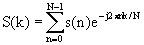(1)

where n =0,1,…….,N-1, and N is the number of samples in the signal s(n). k represents the discrete frequency index and j is equal to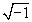. The result of the DFT is a complex-valued sequence of length N.

The IDFT is defined as: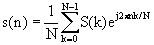, 0≤ n≤ N-1 (2)

The Mel filter bank

In the MFCCs method, the main advantage is that it uses Melfrequency scaling, which approximates quite well the human auditory system. The Mel scale is defined as :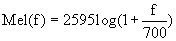(3)

Where Mel is the Mel- frequency scale and f is the frequency on the linear frequency scale.

The DCT

The final stage involves performing a DCT on the log of the Mel spectrum. If the output of the mth Mel filter is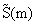, then the MFCCs are given as :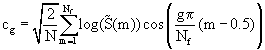(4)

Where g=0,1,…G-1 , G is the number MFCCs, Nf is the number of Mel filters and cg is the gth MFCC. The number of the resulting MFCCs is chosen between 12 and 20, since most of the signal information is represented by the first few coefficients. The 0th coefficient represents the mean value of the input signal.

Discrete transforms can be used for extraction of robust MFCCs in modulation identification systems. The Discrete Wavelet Transform (DWT), the Discrete Cosine Transform (DCT), and the Discrete Sine Transform (DST) have been investigated in the literature for this purpose . Figure 3 illustrates the utilization of discrete transforms in modulation identification systems.

#### Classification

Classification using ANN

Artificial Neural Networks (ANNs) are composed of simple elements operating in parallel. These elements are inspired by biological nervous systems. Typically, neural networks are adjusted, or trained, so that a particular input leads to a specific target output. An ANN usually consists of several layers. Each layer is composed of several neurons or nodes. The connections among each node and the other nodes are characterized by weights. The output of each node is the output of a transfer function, whose input is the summed weighted activity of all node connections. Each ANN has at least one hidden layer in addition to the input and the output layers. In this paper, we use a multi-layer feed-forward neural network .

Figure 4 shows the block diagram of a Multi-Layer Perceptron (MLP). The inputs are fed into the input layer and get multiplied by interconnection weights as they pass from the input layer to the hidden layer, then they get summed and processed by a nonlinear function. Finally, the data is multiplied by interconnection weights, and then processed for the last time within the output layer to produce the neural network output. Mapping is needed to train the neural network.

The training algorithm is mostly defined by the learning rule, that is, the weights update in each training epoch. There are a number of efficient training algorithms for ANNs. Among the most famous is the back-propagation algorithm. An alternative is the back-propagation algorithm with momentum and learning rate to speed up the training. The weight values are updated by a simple gradient descent algorithm.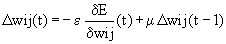(5)

The learning rate, ε, scales the derivative and it has a great influence on the training speed.

In this paper, we consider the resilient back-propagation algorithm . This algorithm performs a direct adaptation of the weight update based on local gradient information.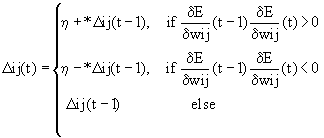(6)

where 0 < η− < 1 < η+. The actual weight update follows a very simple rule as shown in the following equations: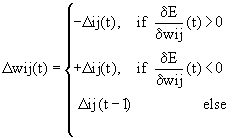(7)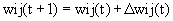(8)

Classification using SVM

SVM is a powerful method for pattern recognition. The method has been applied widely and it has been reported that SVM can perform quite well in many pattern recognition problems .

In this paper, we study the method for multiclass pattern recognition using SVMs. Since the SVM is basically a binary classifier, it is not straight forward to apply it to multiclass recognition problem. We describe the basic theory of the SVM first, and then present the multiclass pattern recognition method based on SVM.

The Support Vector Machine formulation for pattern classification will be described. SVMs were initially developed to perform binary classification; though, applications of binary classification are very limited. Most of the practical applications involve multiclass classification, especially in remote sensing land cover classification. A number of methods have been proposed to implement SVMs to produce multiclass classification. A number of methods to generate multiclass SVMs from binary SVMs have been proposed by researchers and is still a continuing research topic. SVM are based on statistical learning theory and have the aim of determining the location of decision boundaries that produce the optimal separation of classes. In the case of a two-class pattern recognition problem in which the classes are linearly separable the SVM selects from among the infinite number of linear decision boundaries the one that minimizes the generalization error. Thus, the selected decision boundary will be one that leaves the greatest margin between the two classes, where margin is defined as the sum of the distances to the hyperplane from the closest points of the two classes. If the two classes are not linearly separable, the SVM tries to find the hyperplane that maximizes the margin while, at the same time, minimizing a quantity proportional to the number of misclassification errors.

SVM were initially designed for binary (two-class) problems. When dealing with multiple classes, an appropriate multiclass method is needed. Vapnik  suggested comparing one class with the others taken together.

SVM for binary classification: The traditional learning method uses the empirical risk minimization principle, while the statistical learning theory uses the structural risk minimization principle. A learning problem is often defined as follows: suppose a probability measure F (z) in the space Z, consider the function collection Q (z,α ),α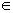Λ, Λ is parameters set. The goal of learning is to minimize the risk function :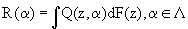(9)

where the probability measure F(z) is unknown, but a certain number of independence samples with the same distribution are given:

Z1,Z2,…,Zl (10)

The goal is to minimize the risk function (5) on the basis of empirical data (6), where Q (z, α) represents a certain loss function. In order to minimize risk function (5) under the unknown distribution function F (z), the following principle is adopted: according to the large number theorem in probability theory, we use arithmetic average as the substitute of the expectation risk R (α).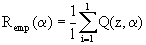(11)

R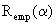, called empirical risk function, is got by (7). We use the function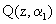, which minimize, to approximate to the function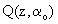that minimizes the risk function R (α), as is known as the Empirical Risk Minimization (ERM). It has been proved by Swami and Sadler  that the minimum empirical risk does not mean the minimum expectation risk. The theorem about the generalizing boundary in statistical learning theory tells us that under the empirical risk minimization principle, the actual risk of learning machines is composed of two parts: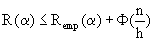(12)

where the first part is called empirical risk of the training samples, the other called confidence interval. The confidence interval is the function of the sets (Q (z, α)) VC-dimension h and the number of the training samples, which monotonic decreases with n/h increasing. We need to minimize the empirical risk and the confidence interval simultaneously, that is Structural Risk Minimization (SRM). The method that implements the Structural Risk Minimization is the Support Vector Machine, and the following is the idea of SVM. In linearly separable case, consider the problem of separating the set of training data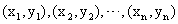into two classes as in figure 5, where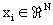is a feature vector and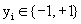is its class label. The linear discriminant function in the N-dimension space is:(13)

If the set can be separated with no error, the function can be expressed as: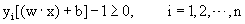(14)

The hyperplanes, which minimize the function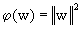subject to (10), are the optimal hyperplanes. The samples, which are both nearest and parallel to the optimal hyperplanes, are called the support vectors. For a training set in order to find the optimal hyperplanes, we need solve a Quadratic programming problem: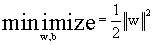(15)

subject to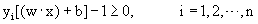(16)

Applying Lagrange multiplier method to solve this optimization problem, we get the optimal classification function: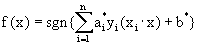(17)

Where sgn(.) is the sign function. In cases where the two classes are non separable, the solution is identical to the separable case with an introduction of relaxation variables . To obtain a linear classifier, one maps the data from the input space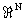to a high dimensional feature space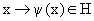, such that the mapped data points of the two classes are linearly separable in the feature space. Assuming there exist a kernel function K such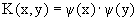, then a nonlinear SVM can be constructed by replacing the inner product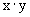in the linear SVM by the kernel function K(x, y), the corresponding classification function is: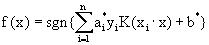(18)

This corresponds to constructing an optimal separating hyperplane in the feature space .

SVM for multiclass classification: Originally, SVMs were developed to perform binary classification. However, applications of binary classification are very limited especially in remote sensing land cover classification where most of the classification problems involve more than two classes. This section provides a brief description of some methods implemented to solve multiclass classification problem with SVM in present study .

One-against-all: The one-against-all method employs c classifiers. The i-th classifier is trained to discriminate between the class wi and all the other classes. During the test phase, the sample is classified using all classifiers and the final decision is made on the basis of the values of the discriminant functions as follows: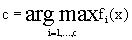(19)

One-against-one: In the case of the one-against-one approach,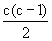classifiers are trained to discriminate between each pair of classes. In order to make the final decision, each classifier votes on one class depending on the sign of its discriminant function. Consequently, the class which collects the highest number of votes is selected.

The kernel is a distance measure in the feature space. For SVM purposes some common kernels are listed in table 1 . For all experiments in this paper we have found that the polynomial kernel function is the best function compared to the others. The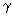-selection could be optimized by fitting a quadratic function on the recognition rates and calculating themax corresponding to the empirical maximum recognition rate.

Function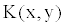Parameters
Linear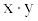none
Polynomial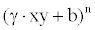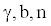Radial basis function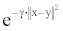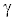Sigmoid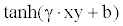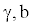Chi-square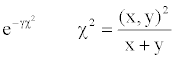Gaussian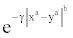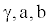Table 1: Common kernel functions for SVM.

#### Results and Discussion

In the training phase of the modulation identification system, a database is first composed for 6 modulation schemes. To generate this database, 6 modulated signals are used to generate MFCCs to form the feature vectors of the database. These features are used to train a SVM. In the testing phase, each one of these modulated signals is transmitted. Similar features to those used in the training are extracted from the noisy modulated signals and used for matching.

The features used in all experiments are 13 MFCCs forming a feature vector of the modulated signal. Seven methods for extracting features are adopted in the experiments. These methods are illustrated follows:

1. The MFCCs are extracted from the modulated signals, only.

2. The features are extracted from the DWT of the modulated signals.

3. The features are extracted from both the original modulated signals and the DWT of these signals and concatenated together.

4. The features are extracted from the DCT of the modulated signals.

5. The features are extracted from both the original modulated signals and the DCT of these signals and concatenated together.

6. The features are extracted from the DST of the modulated signals.

7. The features are extracted from both the original modulated signals and the DST of these signals and concatenated together.

The recognition rate is used as the performance evaluation metric in all experiments. It is defined as the ratio of the number of success identifications to the total number of identification trials.

Recognition rate =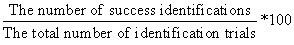(20)

The proposed algorithm in this paper has been verified and validated for various orders of digital modulation types including PSK, MSK, FSK, and QAM. Table 2 illustrates the system specifications. The performance of the classifier has been examined for 100 realizations of each modulation type/order, and the results are presented in tables 6-9 for the different feature extraction methods. If we compare these results with results in tables 3,4 and 5 as in  it is clear that the performance of SVM achieves high recognition rate at low signal to noise ratio and CPU time is less than ANN using machine with specifications (CPU 2.53 GHz PC running with Matlab 7.1).

Parameter Value
Symbol rate 250000 symbol/sec
FTT length 256
No. of carrier 128
No. of parallel channel 256
No. of data 6
Length of guard interval 32
Modulation types QAM, FSK, PSK, MSK

Table 2: System specifications.

SNR(dB) Features from signal Features from DWT Features from signal plus DWT Features from DCT Features from signal plus DCT Features from DST Features from signal plus DST
-10 73.75 86.33 75.58 88.83 95.5 89.58 85.41
-5dB 83.33 95.25 87.91 98 99.25 98.16 96.91
0 90.08 99.66 98.58 100 100 100 99.83
5db 98 100 100 100 100 100 100
10 100 100 100 100 100 100 100
15 100 100 100 100 100 100 100
20 100 100 100 100 100 100 100

Table 3: Recognition rate vs. SNR (dB) for OFDM with AWGN using ANN.

SNR(dB) Features from signal Features from DWT Features from signal plus DWT Features from DCT Features from signal plus DCT Features from DST Features from signal plus DST
-10 80.41 72.08 74.25 80.08 80.83 85 84.91
-5dB 92.66 81.58 79.25 89.75 88.33 94.25 93.08
0 98.75 89.33 93.16 98.91 97.41 99.58 99.16
5db 100 99.58 99.5 100 99.91 100 100
10 100 100 100 100 100 100 100
15 100 100 100 100 100 100 100
20 100 100 100 100 100 100 100

Table 4: Recognition rate vs. SNR (dB) for OFDM with LMMSE using ANN.

SNR(dB) Features from signal Features from DWT Features from signal plus DWT Features from DCT Features from signal plus DCT Features from DST Features from signal plus DST
-10 75.25 71 71.5 75.41 78.25 74.91 79.83
-5dB 80.75 74.08 75.75 82.08 86.83 83 83.16
0 88.33 82.41 84.25 91.25 93.91 91.58 93.25
5db 93.91 89.58 91.75 97.41 98.75 97.16 98.08
10 97.91 97.08 96.91 99 99.58 99.41 99.58
15 99.25 98.91 99.41 99.91 99.83 99.91 100
20 99.5 99.91 99.83 100 100 100 100

Table 5: Recognition rate vs. SNR (dB) for OFDM with ZF using ANN.

SNR(dB) Features from signal Features from DWT Features from signal plus DWT Features from DCT Features from signal plus DCT Features from DST Features from signal plus DST
-10 99.5 98.83 99.33 98.83 99.33 99.66 99.16
-5 99.33 99.66 99.33 98.83 99.33 98.33 99.33
0 99.33 99.33 99.33 100 99 99.66 98.66
5 99 98.83 99.16 99.33 99 100 99
10 98.5 99.33 98.83 100 99.16 100 99.16
15 98.5 99.33 99.33 100 100 100 100
20 100 100 100 100 100 100 100

Table 6: Recognition rate vs. SNR (dB) in OFDM with AWGN Using SVM.

SNR(dB) Features from signal Features from DWT Features from signal plus DWT Features from DCT Features from signal plus DCT Features from DST Features from signal plus DST
-10 97.83 97.66 97.83 98.83 98.33 98.33 98.5
-5 98 98 97.83 98.33 98.33 98.5 98.5
0 98..5 98.16 98.66 98.83 98.16 99 98.5
5 97.66 98.5 99 100 98.5 99.16 98
10 98.66 98.83 98.33 100 98.83 99.16 99.16
15 98.5 99.33 98.83 100 100 100 99.16
20 100 99.33 99.16 100 100 100 100

Table 7: Recognition rate vs. SNR (dB) in OFDM with LMMSE Using SVM.

SNR(dB) Features from signal Features from DWT Features from signal plus DWT Features from DCT Features from signal plus DCT Features from DST Features from signal plus DST
-10 99.66 99.5 99.5 98.33 99.66 98.33 99.5
-6 99.5 99.66 99.66 98.66 99.5 98.5 99.33
0 99.5 99.33 99.33 100 99.5 99.16 99.16
5 99.33 98.83 99.33 99.83 99.16 98.5 99.66
10 99.33 98.83 99.66 100 99.33 99.33 98.16
15 99.16 100 98.83 100 99.66 100 99.16
20 98.16 100 99.16 100 100 100 100

Table 8: Recognition rate vs. SNR (dB) in OFDM with ZF Using SVM.

Features Processing time/ signal (sec) for all Degradations
OFDM with AWGN OFDM with LMMSE OFDM with ZF
SVM ANN SVM ANN SVM ANN
Features from signal 0.03 0.5 0.05 0.5 0.03 0.5
Features from DWT 0.04 0.1 0.01 0.1 0.03 0.2
Features from signal plus DWT 0.04 0.2 0.02 0.2 0.03 0.2
Features from DCT 0.05 0.27 0.05 0.2 0.05 0.25
Features from signal plus DCT 0.05 0.3 0.03 0.37 0.04 0.3
Features from DST 0.05 0.8 0.05 0.8 0.05 0.75
Features from signal plus DST 0.05 0.2 0.03 0.2 0.03 0.2

Table 9: Comparison between CPU time using SVM and ANN classifiers.

#### Conclusion

This paper has presented a suggested approach for automatic modulation recognition based on using MFCCs for feature extraction and SVM is used as a classifier. Simulation results illustrated that the modulation type and order can be determined by extracting cepstral features from the signals and from their transforms such as DWT, DCT and DST, and classifying these features. Signal classification from the SVM has achieved minimum CPU time and high recognition rate compared with ANN classifier.

#### References

Select your language of interest to view the total content in your interested language

### Article Usage

• Total views: 14864
• [From(publication date):
December-2012 - Nov 21, 2019]
• Breakdown by view type
• HTML page views : 11008Can't read the image? click here to refresh